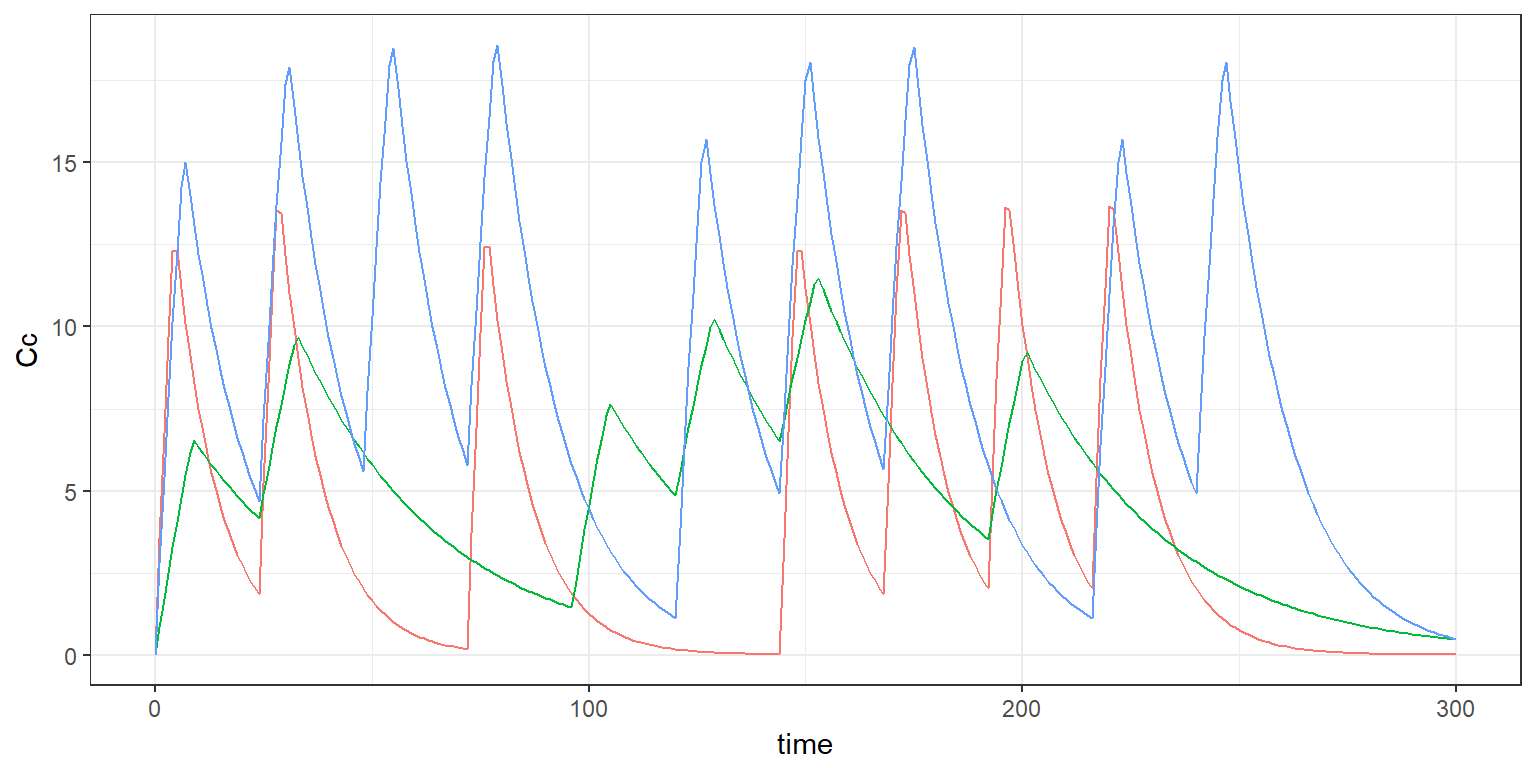$$\newcommand{\esp}{\mathbb{E}\left(#1\right)} \newcommand{\var}{\mbox{Var}\left(#1\right)} \newcommand{\deriv}{\dot{#1}(t)} \newcommand{\prob}{ \mathbb{P}\!(#1)} \newcommand{\eqdef}{\mathop{=}\limits^{\mathrm{def}}} \newcommand{\by}{\boldsymbol{y}} \newcommand{\bc}{\boldsymbol{c}} \newcommand{\bpsi}{\boldsymbol{\psi}} \def\pmacro{\texttt{p}} \def\like{{\cal L}} \def\llike{{\cal LL}} \def\logit{{\rm logit}} \def\probit{{\rm probit}} \def\one{{\rm 1\!I}} \def\iid{\mathop{\sim}_{\rm i.i.d.}} \def\simh0{\mathop{\sim}_{H_0}} \def\df{\texttt{df}} \def\res{e} \def\xomega{x} \newcommand{\argmin}{{\rm arg}\min_{#1}} \newcommand{\argmax}{{\rm arg}\max_{#1}} \newcommand{\Rset}{\mbox{\mathbb{R}}} \def\param{\theta} \def\setparam{\Theta} \def\xnew{x_{\rm new}} \def\fnew{f_{\rm new}} \def\ynew{y_{\rm new}} \def\nnew{n_{\rm new}} \def\enew{e_{\rm new}} \def\Xnew{X_{\rm new}} \def\hfnew{\widehat{\fnew}} \def\degree{m} \def\nbeta{d} \newcommand{\limite}{\mathop{\longrightarrow}\limits_{#1}} \def\ka{k{a}} \def\ska{k{\scriptscriptstyle a}} \def\kel{k{e}} \def\skel{k{\scriptscriptstyle e}} \def\cl{C{\small l}} \def\Tlag{T\hspace{-0.1em}{lag}} \def\sTlag{T\hspace{-0.07em}{\scriptscriptstyle lag}} \def\Tk{T\hspace{-0.1em}{k0}} \def\sTk{T\hspace{-0.07em}{\scriptscriptstyle k0}} \def\thalf{t{1/2}} \newcommand{\Dphi}{\partial_\pphi #1} \def\asigma{a} \def\pphi{\psi} \newcommand{\stheta}{{\theta^\star}} \newcommand{\htheta}{{\widehat{\theta}}}$$

# 1 Introduction

The dosage regimen is the modality of drug administration that is chosen to reach some therapeutic objective. Adherence is defined as the extent to which patients are able to follow the recommendations for prescribed treatments. On the other hand, nonadherence means that the prescribed dosage regimen was not respected by the patient.

We will consider different types of nonadherence:

• A prescribed dose is not taken at all,
• patients may use more or less than the prescribed amount,
• patients may use their medication at the wrong time.

Then, several options are available for simulating nonadherence using simulx.

# 2 Using random individual dosage regimens

We will consider a simple example using a basic PK model. Our objective is to predict the exposure (i.e. to compute the predicted plasmatic concentration) for 3 patients.

N <- 3

myPKmodel1 <- inlineModel("
[LONGITUDINAL]
input={Tk0,V,Cl}

EQUATION:
Cc = pkmodel(Tk0, V, Cl)

[INDIVIDUAL]
input={Tk0_pop,V_pop,Cl_pop,omega_Tk0,omega_V,omega_Cl}

DEFINITION:
Tk0   = {distribution=lognormal,   prediction=Tk0_pop,  sd=omega_Tk0}
V     = {distribution=lognormal,   prediction=V_pop,    sd=omega_V}
Cl    = {distribution=lognormal,   prediction=Cl_pop,   sd=omega_Cl}
")

pk.param   <- c(
Tk0_pop   = 5,    omega_Tk0   = 0.2,
V_pop     = 10,   omega_V     = 0.5,
Cl_pop    = 0.5,  omega_Cl    = 0.2
)

out <- list(name="Cc", time=0:300)

The precribed dosage regimen consists in repeated oral administrations of 100mg every 24 hours:

tdose <- seq(0,240,by=24)
amtdose <- 100
adm1 <- list(amount=amtdose, time=tdose)

We can simulate 3 patients and compute there predicted concentrations, assuming a full adherence to this dosage regimen:

res1 <- simulx(model     = myPKmodel1,
parameter = pk.param,
output    = out,
group     = list(size=N, level="individual"),
settings  = list(seed=12345))

pl1 <- ggplot(res1$Cc) + geom_line(aes(time,Cc,colour=id)) + theme(legend.position = "none") print(pl1)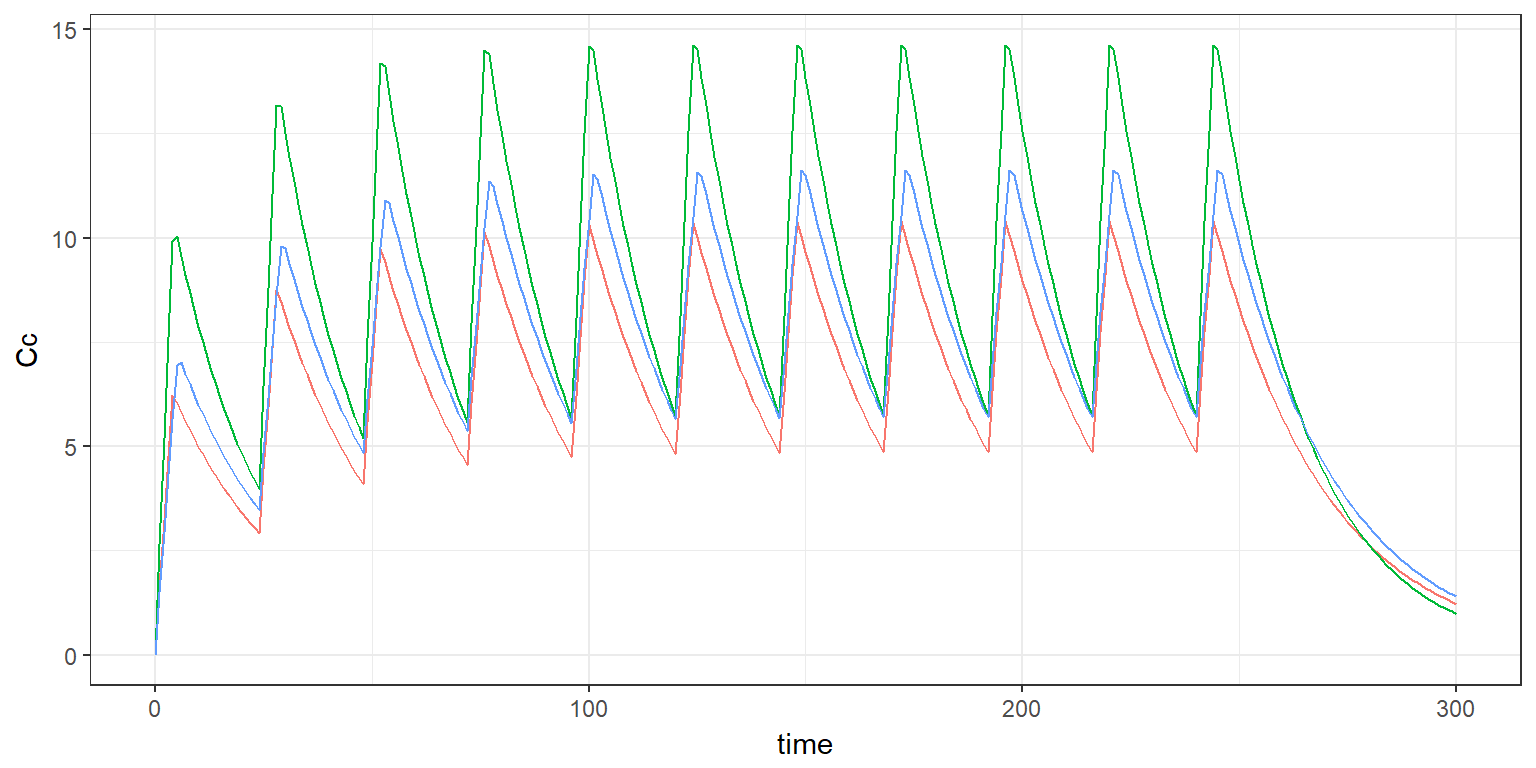Individual dosage regimens could be equivalently defined instead of a global one: #---- individual dosage regimens ---------------------- ndose <- length(tdose) adm2 <- data.frame(id = rep(1:N,each=ndose), amount=amtdose, time=rep(tdose,N)) res2 <- simulx(model = myPKmodel1, parameter = pk.param, treatment = adm2, output = out, settings = list(seed=12345)) Removing a row from the data frame adm2 means that the prescribed dose was not taken. If we assume, for instance, that only 70% of the prescribed doses are taken, we can randomly remove some rows from adm2: #------ non adherence <=> remove treatment lines -------------------- adherence.rate <- 0.7 adm3 <- adm2[runif(N*ndose)<adherence.rate,] res3 <- simulx(model = myPKmodel1, parameter = pk.param, treatment = adm3, output = out, settings = list(seed=12345)) pl3 <- ggplot(res3$Cc) + geom_line(aes(time,Cc,colour=id)) + theme(legend.position = "none")
print(pl3)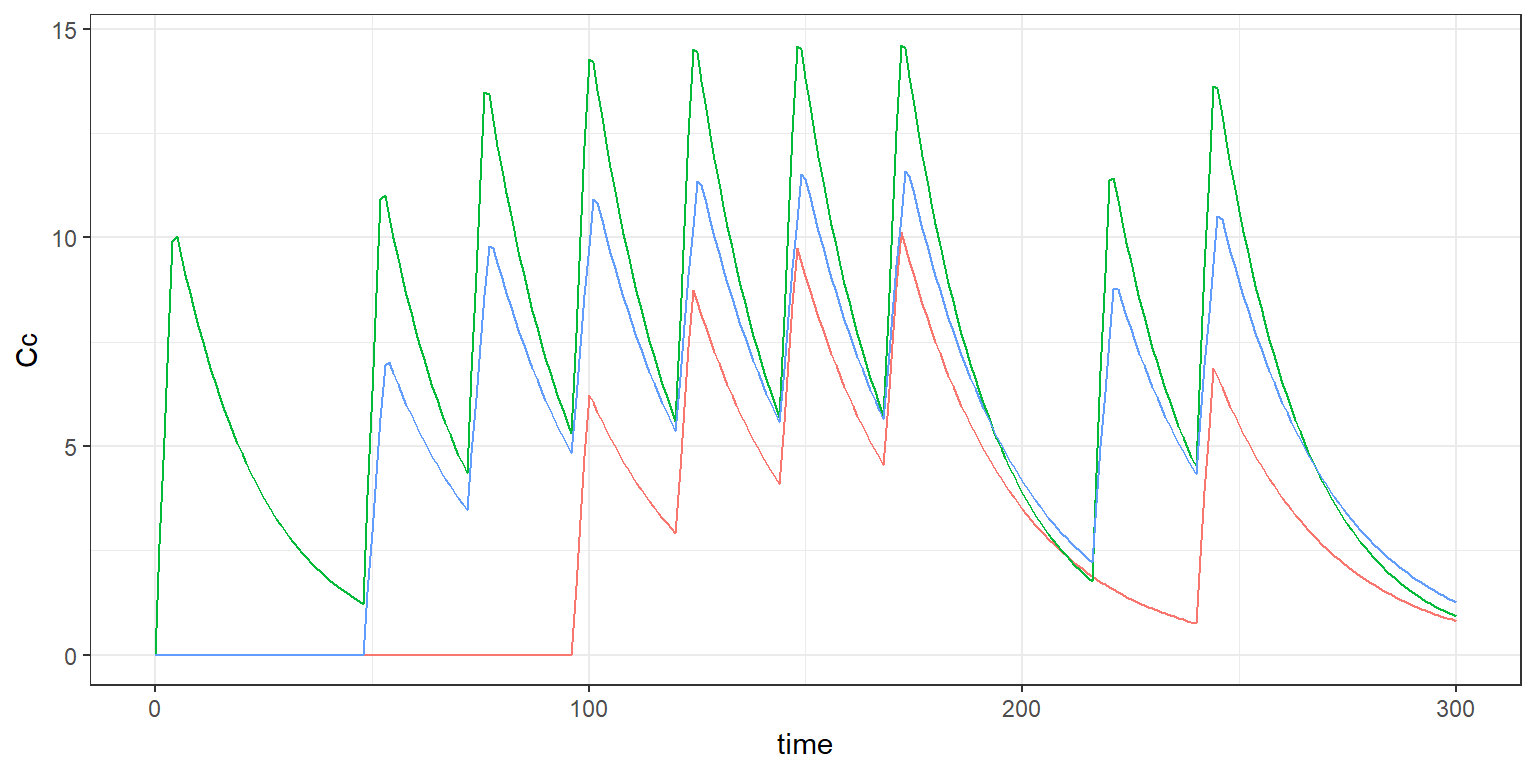If we now assume that the prescribed amounts and times are not exactly respected, we can consider the amount and time as random variables. Normal distributions are used in the following example:

#------- variability on dosing times and amounts ----------------
sd.time <- 3
sd.amount <- 10
adm4$time <- adm4$time + rnorm(N*ndose)*sd.time
adm4$amount <- adm4$amount + rnorm(N*ndose)*sd.amount
head(adm4)
##   id    amount       time
## 1  1 116.32446   1.756586
## 2  1 102.54271  26.128398
## 3  1 104.91188  47.672090
## 4  1  96.75913  70.639508
## 5  1  83.37950  97.817662
## 6  1 117.67734 114.546132
res4 <- simulx(model     = myPKmodel1,
parameter = pk.param,
output    = out,
settings  = list(seed=12345))

pl4 <- ggplot(res4$Cc) + geom_line(aes(time,Cc,colour=id)) + theme(legend.position = "none") print(pl4)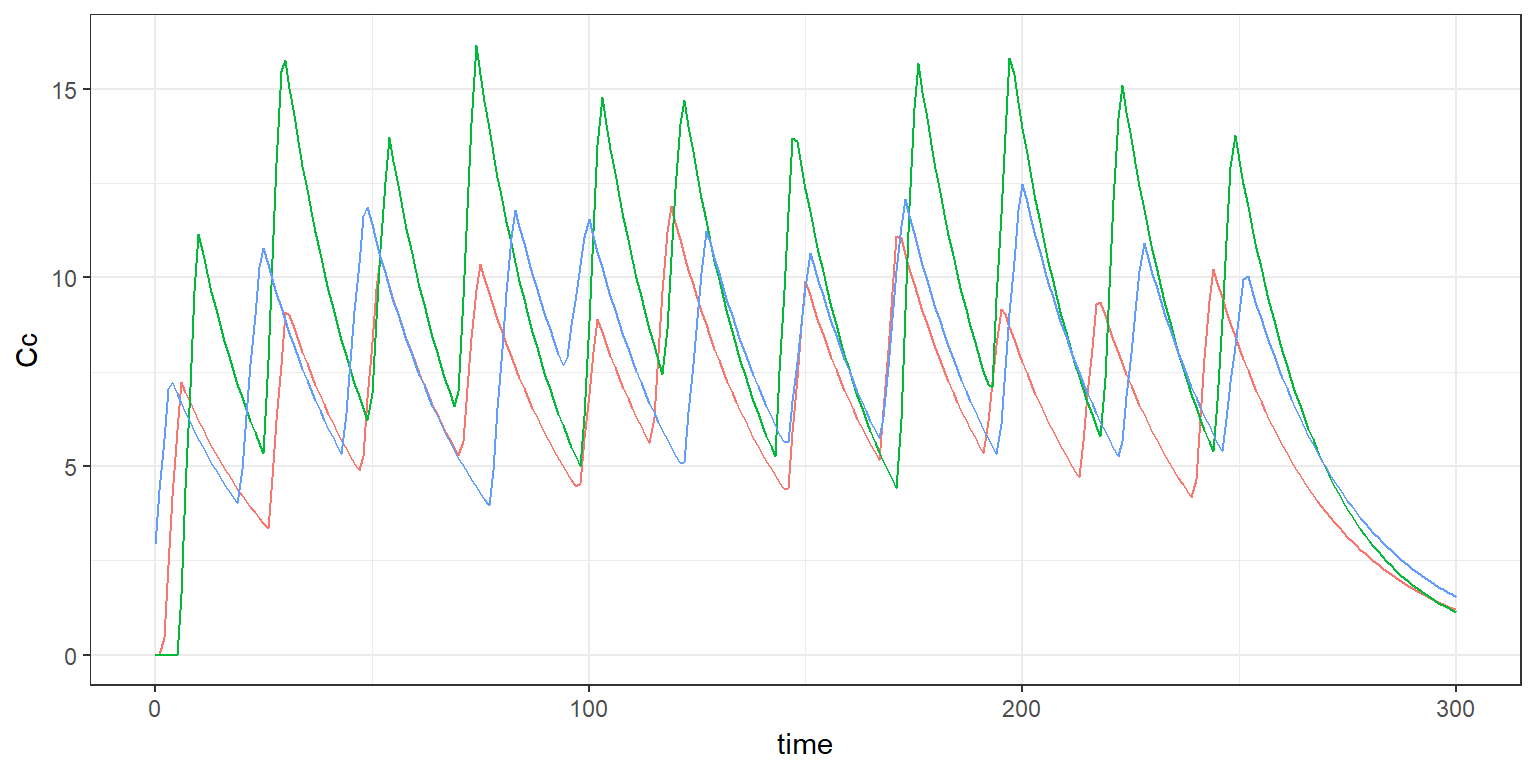# 3 Defining nonadherence as a regression variable We can equivalently define in the structural model the fraction of dose which is effectively administrated myPKmodel2 <- inlineModel(" [LONGITUDINAL] input={Tk0,V,Cl,fd} fd = {use=regressor} EQUATION: Cc = pkmodel(Tk0, V, Cl, p=fd) [INDIVIDUAL] input={Tk0_pop,V_pop,Cl_pop,omega_Tk0,omega_V,omega_Cl} DEFINITION: Tk0 = {distribution=lognormal, prediction=Tk0_pop, sd=omega_Tk0} V = {distribution=lognormal, prediction=V_pop, sd=omega_V} Cl = {distribution=lognormal, prediction=Cl_pop, sd=omega_Cl} ") Here, $$fd=1$$ means that the prescribed amount has been taken while $$fd=0$$ means that the dose was not taken at all: set.seed(123) ndose <- length(tdose) fd <- adm2[-2] fd$fd=as.numeric(runif(N*ndose)<adherence.rate)
head(fd)
##   id time fd
## 1  1    0  1
## 2  1   24  0
## 3  1   48  1
## 4  1   72  0
## 5  1   96  0
## 6  1  120  1
res5 <- simulx(model     = myPKmodel2,
parameter = pk.param,
output    = out,
regressor = fd,
settings  = list(seed=12345))

pl5 <- ggplot(res5$Cc) + geom_line(aes(time,Cc,colour=id)) + theme(legend.position = "none") print(pl5)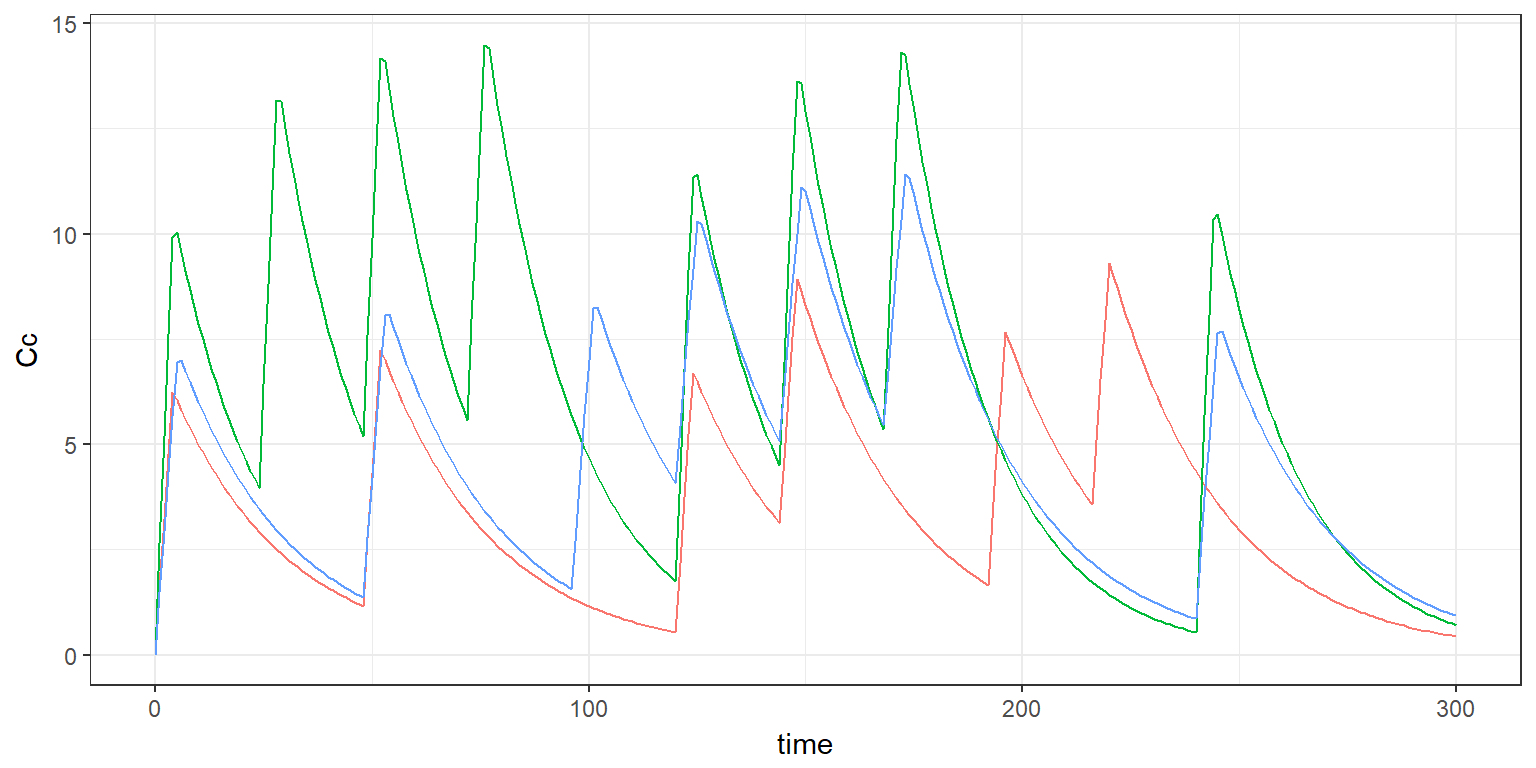On the other hand, $$fd\neq 1$$ means that the prescribed amount was not respected. set.seed(123) fd$fd=adm2$amount*rnorm(ndose,1,0.1) head(fd) ## id time fd ## 1 1 0 94.39524 ## 2 1 24 97.69823 ## 3 1 48 115.58708 ## 4 1 72 100.70508 ## 5 1 96 101.29288 ## 6 1 120 117.15065 fd <- data.frame(id = rep(1:N,each=ndose), time=rep(tdose,N), fd=as.numeric(runif(N*ndose)<adherence.rate)) res6 <- simulx(model = myPKmodel2, parameter = pk.param, treatment = adm1, output = out, regressor = fd, settings = list(seed=12345)) pl6 <- ggplot(res6$Cc) + geom_line(aes(time,Cc,colour=id)) + theme(legend.position = "none")
print(pl6)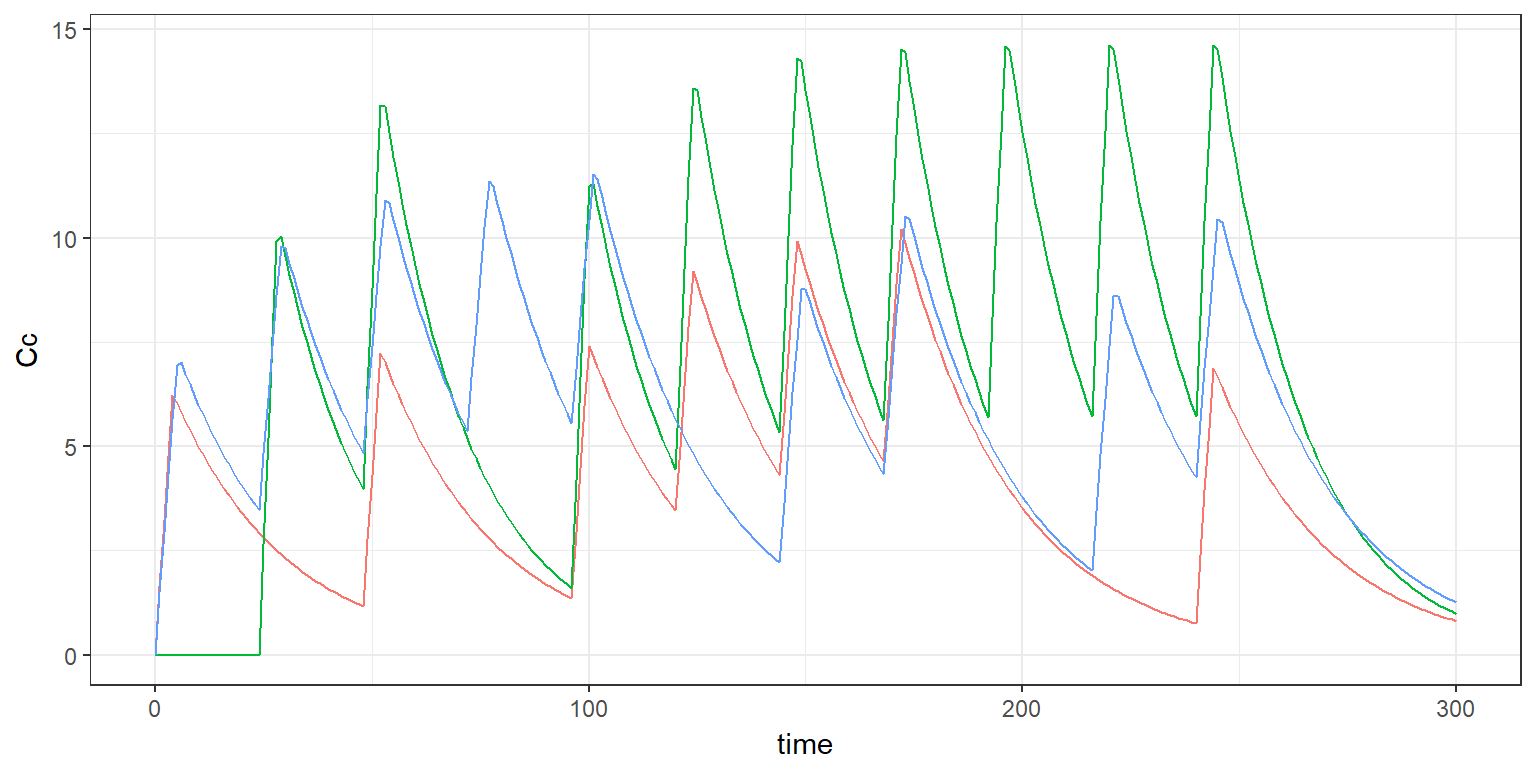# 4 Defining nonadherence as a sequence of individual parameters

The fraction of dose which is effectively administrated can be alternatively defined as a sequence of individual parameters in the model itself. If we assume that this fraction may vary from a dose to another one for a given patient, an occasion should be defined for each dose and inter occasion variability (IOV) should be introduced for this parameter.

In the following example, a logitnormal distribution is used in order to constraint this fraction to be between 0 and 1 (any other distribution for positive random variables could be used):

myPKmodel3a <- inlineModel("
[LONGITUDINAL]
input={Tk0,V,Cl,fd}

EQUATION:

Cc = pkmodel(Tk0, V, Cl, p=fd)

[INDIVIDUAL]
input={Tk0_pop,V_pop,Cl_pop,fd_pop,omega_Tk0,omega_V,omega_Cl,gamma_fd}

DEFINITION:
Tk0   = {distribution=lognormal,   prediction=Tk0_pop,  sd=omega_Tk0}
V     = {distribution=lognormal,   prediction=V_pop,    sd=omega_V}
Cl    = {distribution=lognormal,   prediction=Cl_pop,   sd=omega_Cl}
fd    = {distribution=logitnormal,      prediction=fd_pop, varlevel={id, id*occ}, sd={0,gamma_fd}}
")

Setting $$fd_{\rm pop}=1$$ and $$\gamma_{fd}=0$$ means that all the prescribed doses are correctly taken.

In the following example, this fraction is randomly distributed around 0.8:

occ  <- list(time=tdose, name="occ")
fd.param <- c(fd_pop=0.8, gamma_fd=1)
res7 <- simulx(model     = myPKmodel3a,
parameter = list(pk.param, fd.param),
varlevel  = occ,
output    = list(out, list(name="fd")),
group     = list(size=N, level="individual"),
settings  = list(seed=12345))

head(res7$parameter.iov) ## id time occ fd ## 1 1 0 1 0.8547146 ## 2 1 24 2 0.7223659 ## 3 1 48 3 0.7384445 ## 4 1 72 4 0.3859724 ## 5 1 96 5 0.7956514 ## 6 1 120 6 0.8886648 pl7 <- ggplot(res7$Cc) + geom_line(aes(time,Cc,colour=id)) + theme(legend.position = "none")
print(pl7)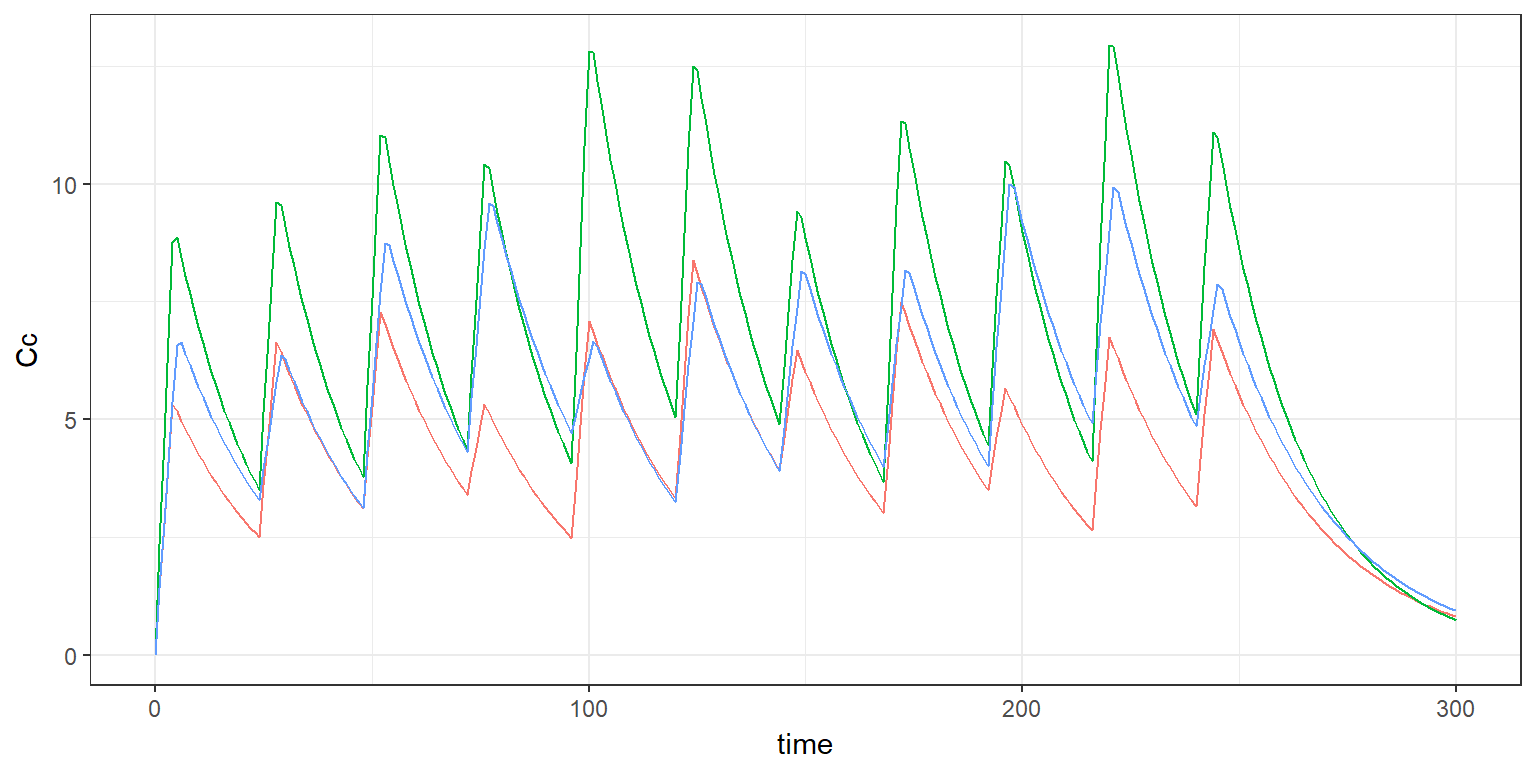Considering that some doses are not taken at all requires to convert a continuous random variable into a Bernoulli one:

myPKmodel3b <- inlineModel("
[LONGITUDINAL]
input={Tk0,V,Cl,z,zlim}

EQUATION:
if z<zlim
p = 1
else
p =0
end
Cc = pkmodel(Tk0, V, Cl, p)

[INDIVIDUAL]
input={Tk0_pop,V_pop,Cl_pop,omega_Tk0,omega_V,omega_Cl}

DEFINITION:
Tk0   = {distribution=lognormal,   prediction=Tk0_pop,  sd=omega_Tk0}
V     = {distribution=lognormal,   prediction=V_pop,    sd=omega_V}
Cl    = {distribution=lognormal,   prediction=Cl_pop,   sd=omega_Cl}
z     = {distribution=normal,      prediction=0, varlevel={id, id*occ}, sd={0,1}}
")

out.p <- list(name=c("p"), time=occ$time) res8 <- simulx(model = myPKmodel3b, parameter = list(pk.param, adh.param), treatment = adm1, varlevel = occ, output = list(out, out.p), group = list(size=N, level="individual"), settings = list(seed=1234)) head(res8$p)
##   id time p
## 6  1  120 0
pl8 <- ggplot(res8\$Cc) + geom_line(aes(time,Cc,colour=id)) + theme(legend.position = "none")
print(pl8)